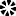## What kind of math is used in electrical engineering?

What kind of math is used in electrical engineering?

Electrical engineers design, develop, test, and supervise the manufacture of electrical equipment. Some of this equipment includes electric motors, machinery controls, lighting and wiring in buildings, vehicles, aircraft, radar and navigation systems, and power generation, control and transmission devices used by electric utilities.

Engineers typically enter the occupation with a bachelor’s degree in mathematics or an engineering specialty, but some basic research positions may require a graduate degree. Most engineering programs involve a concentration of study in an engineering specialty, along with courses in both mathematics and the physical and life sciences. Engineers offering their services directly to the public must be licensed. Continuing education to keep current with rapidly changing technology is important for engineers.

Mathematics is a big part of an engineer's daily work, including statistics, calculus, algebra, geometry and trigonometry. The type of math an engineer uses will depend on the type of engineer she/he is and the type of project in which they are involved.

Below is a list of math used in electrical engineering:

Algebra

The basic algebra students learn in high school is only the beginning, a necessary foundation for almost any further development in either mathematics or electrical engineering. A prospective engineering student must be able to solve variable equations and understand how to factor complex polynomial expressions. You must be very comfortable working with logarithms and be familiar with algebraic manipulation of complex numbers — numbers with both real and imaginary roots. You will also need to be familiar with Boolean algebra, a logical system that is central to understanding circuit design.

Trigonometry

Trigonometry is the science of measuring triangles. Engineers may use plane trigonometry to determine the size of an irregularly shaped parcel of land. It may also be used or to determine the height of an object based solely on the distance to the object and the angle, up or down, from the observer. Spherical trigonometry is used by naval engineers in ship design and by mechanical engineers working on such arcane projects as the design of mechanical hand for an underwater robot.

Geometry

Analytic geometry uses numerical techniques to describe classic geometric concepts including lines, points and curves. While the ancient Greeks understood geometric concepts of conic sections, parabolas, circles, etc., analytic geometry allows for deeper analysis of these forms. It describes these ancient geometric constructs with equations representing relationships between quantities. Analytic geometry is one of the most significant tools for understanding and describing the relationships between changing quantities, and it is essential for any advanced study in electrical engineering.

Calculus

Calculus is used by engineers to determine rates of change or rates by which factors, such as acceleration or weight, change. It might tell NASA scientists at what point the change in a satellite's orbit will cause the satellite to strike an object in space. A more mundane task for calculus might be determining how large a box must be to accommodate a specific number of things. An engineer who designs packaging, for example, might know that a product of a certain weight must be packaged in groups of no more than 10 because of their weight. Using calculus, he can calculate both the optimum number of objects per box, plus the optimum size of the box.

Statistics

The role of Statistics in Engineering is indispensable. All practicing engineers realise that soon after getting into the workplace. Starting from designing a product, making a finished one and making it work, at every step an engineer needs help of Statistics in some form or other. From rudimentary Statistics of data collection to Advance Probability in Quantum Mechanics and Space science, Aeronautics and Civil, Mechanical and Electrical, Statistics becomes a tool that engineers just cannot afford to ignore.

Want to learn electrical engineering? Read more about the electrical engineering training we offer at London TFE and book on to our electrical engineering courses.